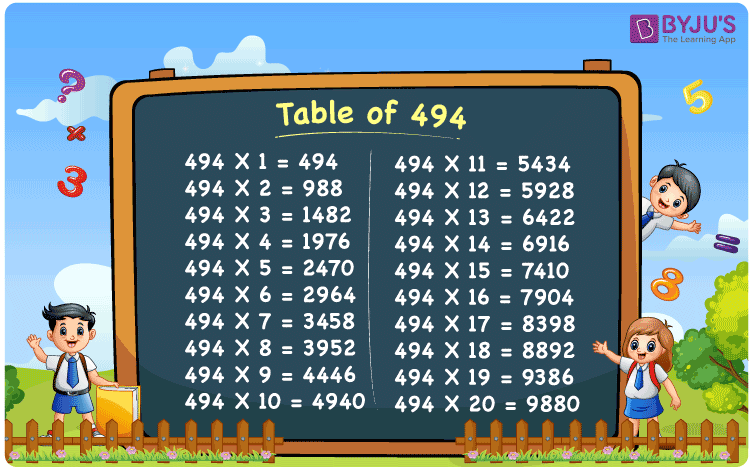Checkout JEE MAINS 2022 Question Paper Analysis : Checkout JEE MAINS 2022 Question Paper Analysis :

# Table of 494

The table of 494 is a multiplication table that includes all multiples of 494. When multiplied by an integer, the 494 times table depicts the repetitive addition of the number 494. When 494 is multiplied by 3, for example, 494 is added three times to itself, resulting in 494 x 3 = 494 + 494 + 494 = 1482. As a result, when 494 is multiplied by various integers, the results change. The Table of 494 can be obtained by multiplying it with positive integers in a row.

## Table of 494 Chart## What is the 494 Times Table?

The table of 494, as previously stated, represents the repetitive addition of the number 494 when multiplied by any other number. Let’s look at how to write the 494 times table.

 494×1 = 494 494 494×2 = 988 494 + 494 = 988 494×3 = 1482 494 + 494 + 494 = 1482 494×4 = 1976 494 + 494 + 494 + 494 = 1976 494×5 = 2470 494 + 494 + 494 + 494 + 494 = 2470 494×6 = 2964 494 + 494 + 494 + 494 + 494 + 494 = 2964 494×7 = 3458 494 + 494 + 494 + 494 + 494 + 494 + 494 = 3458 494×8 = 3952 494 + 494 + 494 + 494 + 494 + 494 + 494 + 494 = 3952 494×9 = 4446 494 + 494 + 494 + 494 + 494 + 494 + 494 + 494 + 494 = 4446 494×10 = 4940 494 + 494 + 494 + 494 + 494 + 494 + 494 + 494 + 494 + 494 = 4940

## Multiplication Table of 494

See the 494-table below, up to 20 times. This table can be used to solve problems involving multiplication.

 494 × 1 = 494 494 × 2 = 988 494 × 3 = 1482 494 × 4 = 1976 494 × 5 = 2470 494 × 6 = 2964 494 × 7 = 3458 494 × 8 = 3952 494 × 9 = 4446 494 × 10 = 4940 494 × 11 = 5434 494 × 12 = 5928 494 × 13 = 6422 494 × 14 = 6916 494 × 15 = 7410 494 × 16 = 7904 494 × 17 = 8398 494 × 18 = 8892 494 × 19 = 9386 494 × 20 = 9880

## Solved Example on the Table of 494

Q. Find the value of 494 x 4 + 100.

Solution: Given,

494 x 4 + 100

= 1970 + 100

= 2070

## Frequently Asked Questions on the Table of 494

### What is the table of 494?

The table of 494 is a multiplication table that shows how many times a number has been multiplied 494 times. It aids in the execution of mathematical operations.

### What is 494 times 3?

The value of 494 times 3 is 494 × 3 = 1482.

### 494 times of which number is equal to 9880?

From the table of 494, 494 times 20 is equal to 9880.
494 x 20 = 9880